# Structured Types

## Modelling Structured Types

The Event-B mathematical language currently does not support a syntax for the direct definition of structured types such as records or class structures. Nevertheless it is possible to model structured types using projection functions to represent the fields/attributes. For example, suppose we wish to model a record structure C with fields e and f (with type E and F respectively). Let us use the following syntax for this (not part of Event-B syntax):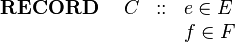$\begin{array}{lcl} \textbf{RECORD}~~~~ C &::& e\in E\\ && f \in F \end{array}$

We can model this structure in Event-B by introducing (in a context) a carrier set C and two functions e and f as constants as follows: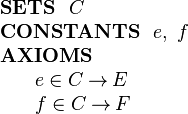$\begin{array}{l} \textbf{SETS}~~ C\\ \textbf{CONSTANTS}~~ e,~ f\\ \textbf{AXIOMS}\\ ~~~~\begin{array}{l} e \in C \tfun E\\ f \in C \tfun F\\ \end{array} \end{array}$

Now, given an element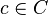$c\in C$ representing a record structure, we write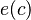$e(c)$ for the e component of c and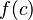$f(c)$ for the f component of c.

E and F can be any type definable in Event-B, including a type representing a record structure.